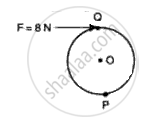Advertisement Remove all ads

# A Wheel of Diameter 3 M is Shown in Fig. 2 with Axle at 0. a Force F = 8 N is Applied at Q in the Direction Shown in Figure. Calculate the Moment of Force About: (I) Centre O, and (Ii) Point P. - Physics

Numerical

A wheel of diameter 3 m is shown in fig. 2 with axle at 0. A force F = 8 N is applied at Q in the direction shown in figure. Calculate the moment of force about:
(i)    centre o, and
(ii)    point p.Advertisement Remove all ads

#### Solution

(i) Moment of force = applied force x perpendicular distance from the line of action

Moment of force at O = 8 x 1.5 = 12 Nm

(ii) Momemnt of force = applied force x perpendicular distance from the line of line

Moment of force at P = 8 x 3 = 24 Nm

Concept: Moment (Turning Effect) of a Force Or Torque
Is there an error in this question or solution?
Advertisement Remove all ads

#### APPEARS IN

Frank ICSE Class 10 Physics Part 2
Chapter 1 Force, Work, Energy and Power
Exercise | Q 8 | Page 57
Advertisement Remove all ads
Advertisement Remove all ads
Share
Notifications

View all notifications

Forgot password?